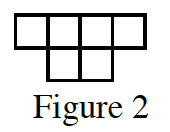### Home > CCA > Chapter 2 > Lesson 2.1.2 > Problem2-22

2-22.

Figure $2$ of a tile pattern is shown at right.  If the pattern grows linearly and if Figure $5$ has $15$ tiles, then find a rule for the pattern.

Make a table relating the figure number to the number of tiles, or write a proportion.

$y=3x$